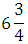# 3/8 is what part of 1/12

1.   3/7

2.  1/12

3.  4/3

4.  None of these

4

None of these

Explanation :
No Explanation available for this question

# The smallest fraction which should be subtracted from the sum ofto make the result a whole number is:

1.  5/12

2.  7/12

3.  1/2

4.  7

4

5/12

Explanation :
No Explanation available for this question

# If x is a positive number, then which of the following fractions has the greatest value

1.  x/x

2.  x/x+1

3.  x+1/x

4.  x+2/x+3

4

x+1/x

Explanation :
No Explanation available for this question

# By how much is three-fifth of 350 greater than four-seventh of 210

1.  95

2.  110

3.  120

4.  210

5.  None of these

5

None of these

Explanation :
No Explanation available for this question

# By how much doesexceed

1.

2.

3.

4.

4Explanation :
No Explanation available for this question

# If 4/5 of an estate be worth Rs. 16,800, then the value of 3/7 of the estate is:

1.  Rs. 9000

2.  Rs. 21,000

3.  Rs. 72,000

4.  Rs. 90,000

4

Rs. 9000

Explanation :
No Explanation available for this question

# Two-fifth of one-fourth of three-seventh of a number is 15. What is half of that number

1.  94

2.  96

3.  188

4.  196

5.  None of these

5

None of these

Explanation :
No Explanation available for this question

# One-fifth of a number exceeds one-seventh of the same by 10. The number is:

1.   125

2.  150

3.  175

4.  200

4

175

Explanation :
No Explanation available for this question

# If x*y=x2 + y2 – xy, then the value of 9*11 is:

1.  93

2.  103

3.  113

4.  121

4

103

Explanation :
No Explanation available for this question

1.   -3

2.  -1.5

3.  -1

4.  2/3

4